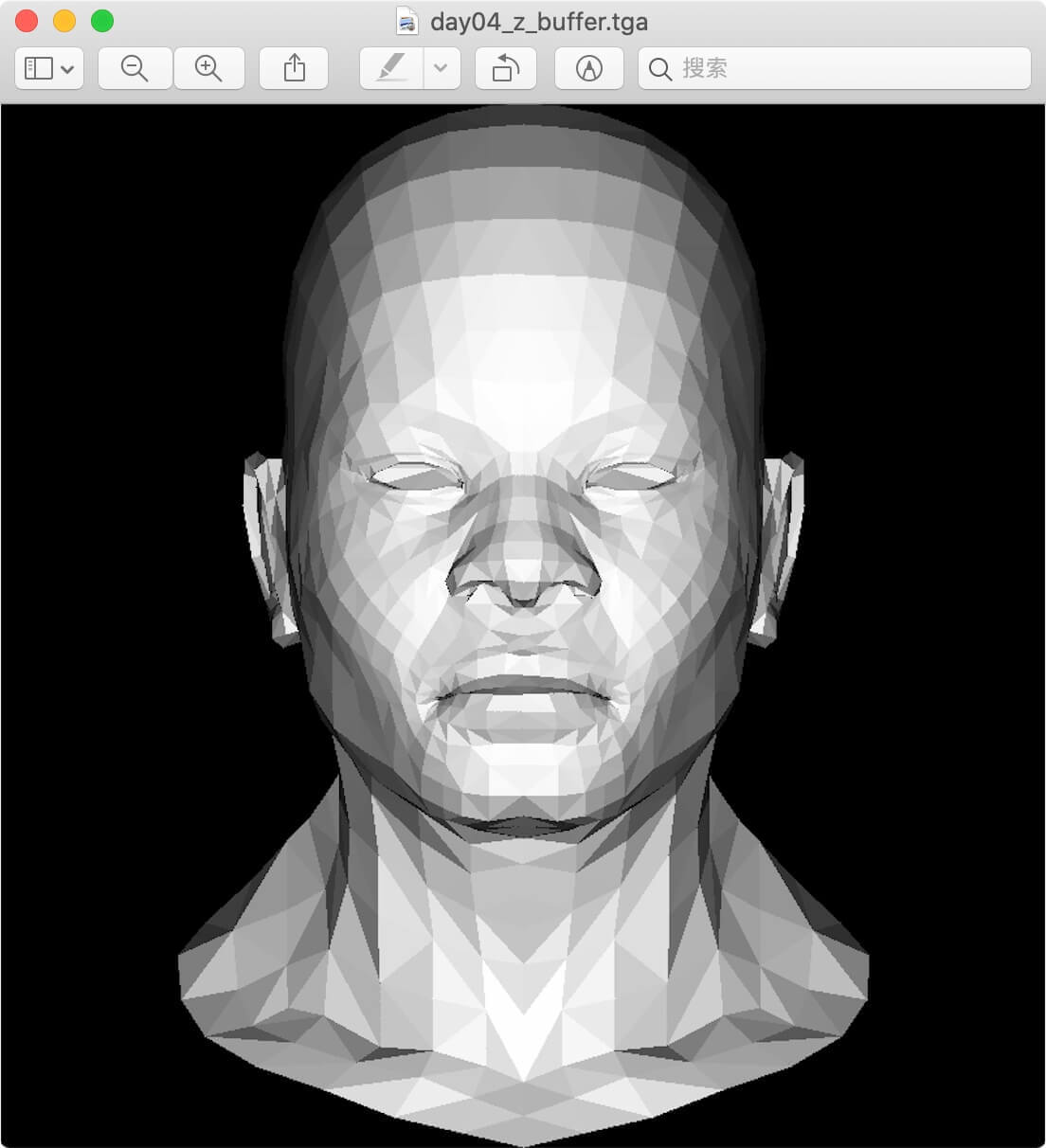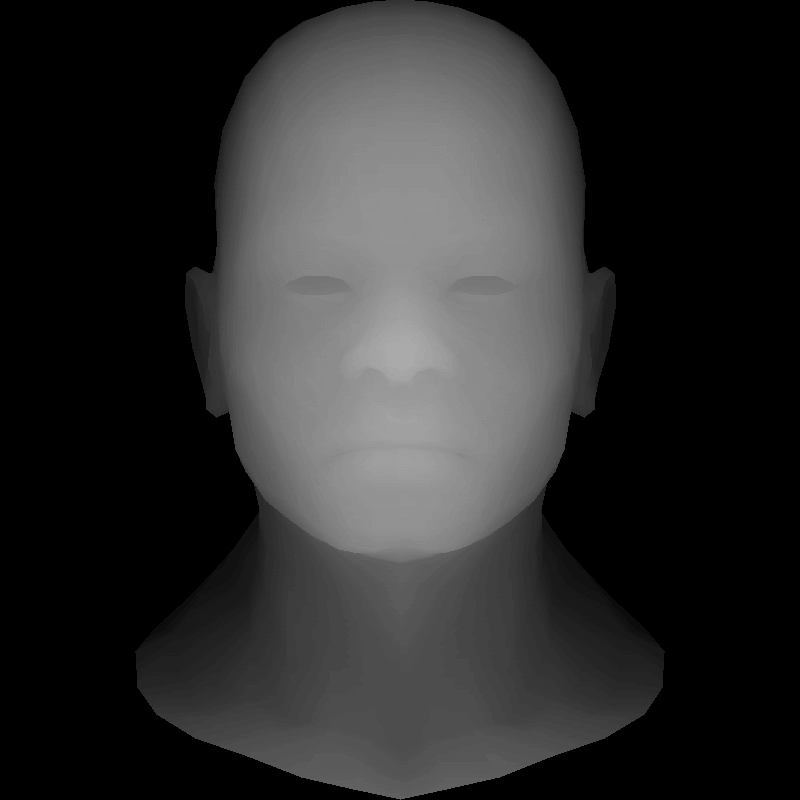# day4：Z-buffering（深度缓冲）

## 1.画家算法#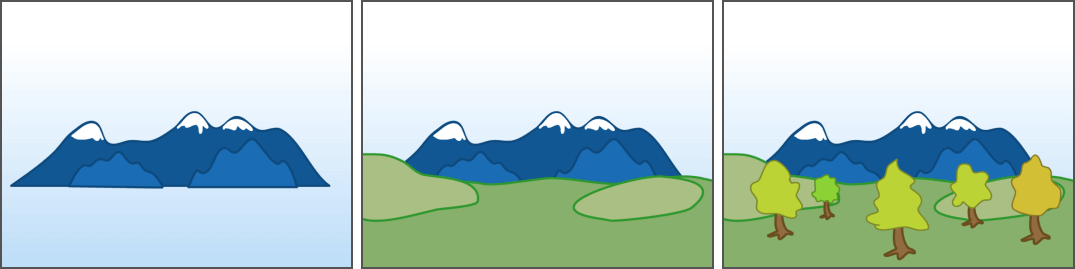• 首先画最远处的山
• 然后画次远处的草原
• 最后画最近的树木

• 首先画 z-index=1 处的山
• 然后画 z-index=2 处的草原
• 最后画 z-index=3 的树木

• 网页通过 CSS 的 z-index 控制层级顺序
• iOS 通过 layer.zPosition 控制层级顺序
• Android 通过 index 控制层级顺序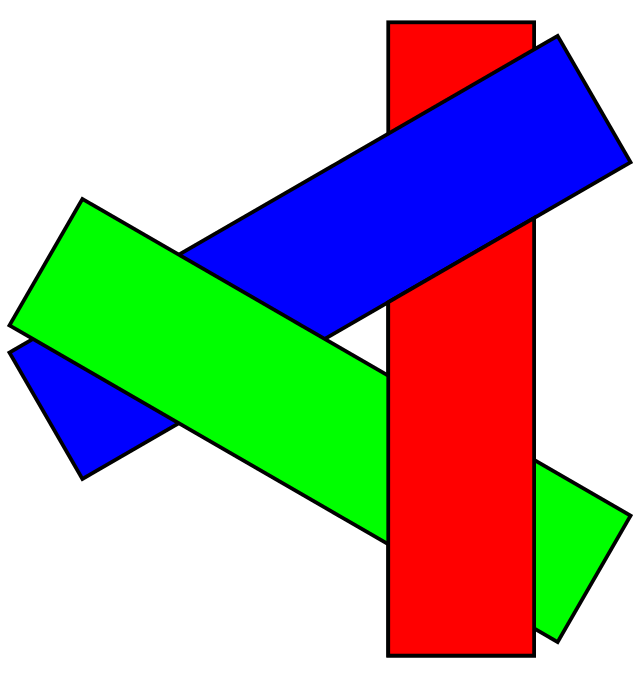## 2.Z-buffering#

Z-buffering，中文名又为「深度图」「深度缓冲」，它是通过记录比较每个像素的深度信息来解决层级问题。

Z-buffering 算法理解起来其实是非常直观的，我们这里借用《虎书 4》里的一张插图（可以关注🛰️号「卤蛋实验室」后台回复「图形学」领取本书）来讲解一下 Z-buffering 的工作原理。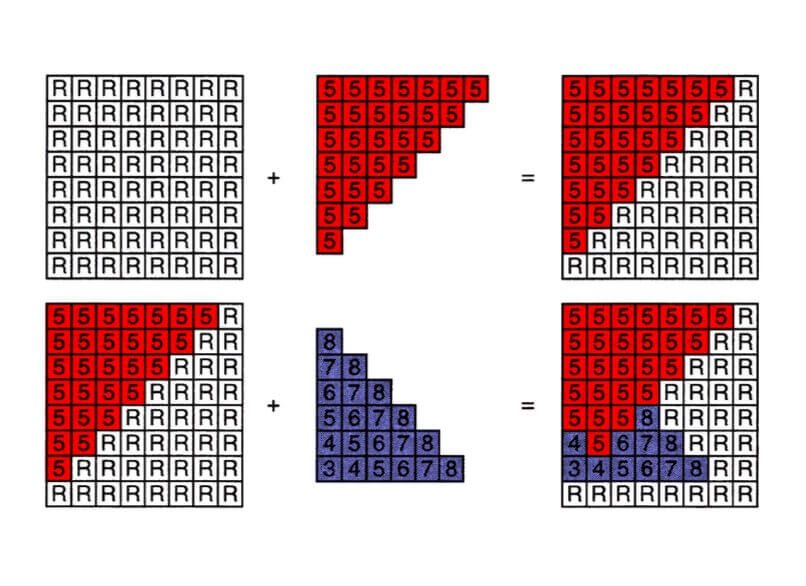// 首先假设深度默认值都是负无穷 -∞(这里可以是无穷大，也可以是无穷小，依坐标系而定)
for (each triangle T) // 遍历每个三角形
for (each sample (x,y,z) in T) // 遍历三角形里的每个像素
if (z > zbuffer[x,y]) // 如果深度大于已有的值，
framebuffer[x,y] = rgb; // 则更新颜色，
zbuffer[x,y] = z; // 并更新 zbuffer
else
// do nothing // 小于已有的值，就说明这个像素点被遮挡不需要绘制了

## 3.代码实现#

// [[1, 2, 3],
// [4, 5, 6],
// [7, 8, 9]]

// [[1, 2, 3], [1, 2, 3,
// [4, 5, 6], => 4, 5, 6,
// [7, 8, 9]] 7, 8, 9],

float *zbuffer = new float[width * height];
for (int i=0; i < width * height; i++) {
zbuffer[i] = -std::numeric_limits<float>::max();
}

//......
Vec3f P;
for (P.x = boxmin.x; P.x <= boxmax.x; P.x++) {
for (P.y = boxmin.y; P.y <= boxmax.y; P.y++) {
Vec3f bc_screen = barycentric(pts, P); // bc 是 Barycentric Coordinates 的缩写
//......
// 计算当前像素的 zbuffer
P.z = 0;
for (int i = 0; i < 3; i++) {
P.z += pts[i] * bc_screen[i];
}
// 更新总的 zbuffer 并绘制
if(zbuffer[int(P.x + P.y * width)] < P.z) {
zbuffer[int(P.x + P.y * width)] = P.z;
image.set(P.x, P.y, color);
}
}
}
//......